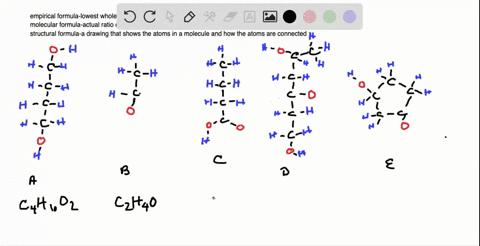🎉 The Study-to-Win Winning Ticket number has been announced! Go to your Tickets dashboard to see if you won! 🎉View Winning Ticket### Which of the following models represent compounds…

04:13University of Maine
Problem 110

# The following scenes represent a chemical reaction between $\mathrm{AB}_{2}$ and $\mathrm{B}_{2}$ :(a) Write a balanced equation for the reaction. (b) What is the limiting reactant? (c) How many moles of product can be made from 3.0 $\mathrm{mol}$ of $\mathrm{B}_{2}$ and 5.0 $\mathrm{mol}$ of $\mathrm{AB}_{2} ?(\mathrm{d})$ How many moles of excess reactant remain after the reaction in part (c)?

## Discussion

You must be signed in to discuss.

## Video Transcript

we can use this picture representing a chemical reaction to look at both with the overall reaction is as well as the idea of a limiting an excess free agent. When we start, we have two different react. It's on this side. If we call our green circles be, we can see that there are molecules of B two. The's react with molecules that have to bees in an A, which will call a need to. So our reactions are a B two plus B two, and then when we look at the product side, we see would make a new compound A with three bees. There are also some B two's still on the side. We can count how many of each there are. So there's 123456 12345 Btu's. And on this side we have a total of six A B threes and to be twos. We can simplify this by combining the B two since they appear on both sides or the equation ist six a B two plus three B two make six a B three, and then finally we can reduce it to the lowest whole number ratio or dividing everything by three to a B two plus B two makes two, maybe three. So this is the overall equation for the chemical reaction that we see to identify which react and is limiting. We can also look at which one we have extra and you can see that we start off with B two and they're still be, too, on the other side. So that means that that's not all reacted where we say it's an excess. So what that means is the one determining our amount of product is a be two or limiting, and we can verify this river calculation using the mole ratios from the equation. If I have six moles of a B two, I should produce according to the equation two moles of a B three for every two moles of a B two or six moles of product. If I have five moles of be to the other reactor, I know that I'll produce two moles of a B three for every one mole of B two from the coefficients of the balanced equation, or I'll produce 10 moles, maybe three. Since six is less than 10 that means that a B two is the limiting re agent. We can also use the information for different quantities besides those given in the picture. So if, for example, I have three moles of B two and five moles of a B two, I can predict how much product for each one I predict. I calculate the product like I did above so three moles of B two, and I know that there are using the mole ratio two moles of a B three for every one mole would be to. So I should produce six malls. Be to if I have Dr Moles of a B two. According to my balanced equation, there will be two moles of a B three for every two moles of a B two or produce. Five moles have a B three. Since five is less than six, that's the amount of product that I'll produce. That means that I have excess free to. So if I were to produce five moles of a B three, I only need half assed much be too or 2.5 malls be to, but I have three, so three minus 2.5 indicates that I'll have 0.5 moles be to in excess were left over